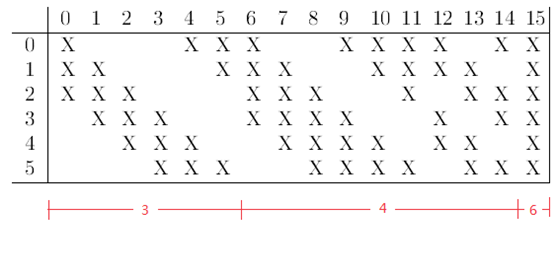# Group convolution in pytorch

Hello everyone, I have a question that I have been puzzled for a long time.
In the conv2d function, only the arg “group” controls the group convolution. But what can i do to specify some specific channels for convolution? For examole, the convolution layer connections of lenet shown in the following picture.Someone can help me？ Thanks.Could you explain the image a bit and what the axes and labels stand for?

Each column represents a convolution kernel(output channels) and each row represents an input channel. In this conv layer the input channel number is 6 and the output channel number is 16.The label “x” represents the connections. For example, the outout channel 0 is connected with input channel 0,1 and 2.